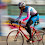### C C++ CODE : LU Decomposition for solving linear equations

Working C C++  Source code program for LU Decomposition for solving linear equations
``````/************** LU Decomposition for solving linear equations ***********/
#include<iostream.h>
#include<conio.h>
int main()
{
int n,i,k,j,p;
float a,l={0},u={0},sum,b,z={0},x={0};
clrscr();
cout<<"Enter the order of matrix ! ";
cin>>n;
cout<<"Enter all coefficients of matrix : ";
for(i=1;i<=n;i++)
{
cout<<"\nRow "<<i<<"  ";
for(j=1;j<=n;j++)
cin>>a[i][j];
}
cout<<"Enter elements of b matrix"<<endl;
for(i=1;i<=n;i++)
cin>>b[i];
//********** LU decomposition *****//
for(k=1;k<=n;k++)
{
u[k][k]=1;
for(i=k;i<=n;i++)
{
sum=0;
for(p=1;p<=k-1;p++)
sum+=l[i][p]*u[p][k];
l[i][k]=a[i][k]-sum;
}

for(j=k+1;j<=n;j++)
{
sum=0;
for(p=1;p<=k-1;p++)
sum+=l[k][p]*u[p][j];
u[k][j]=(a[k][j]-sum)/l[k][k];
}
}
//******** Displaying LU matrix**********//
cout<<endl<<endl<<"LU matrix is "<<endl;
for(i=1;i<=n;i++)
{
for(j=1;j<=n;j++)
cout<<l[i][j]<<"  ";
cout<<endl;
}
cout<<endl;
for(i=1;i<=n;i++)
{
for(j=1;j<=n;j++)
cout<<u[i][j]<<"  ";
cout<<endl;
}

//***** FINDING Z; LZ=b*********//

for(i=1;i<=n;i++)
{                                        //forward subtitution method
sum=0;
for(p=1;p<i;p++)
sum+=l[i][p]*z[p];
z[i]=(b[i]-sum)/l[i][i];
}
//********** FINDING X; UX=Z***********//

for(i=n;i>0;i--)
{
sum=0;
for(p=n;p>i;p--)
sum+=u[i][p]*x[p];
x[i]=(z[i]-sum)/u[i][i];
}
//*********** DISPLAYING SOLUTION**************//
cout<<endl<<"Set of solution is"<<endl;
for(i=1;i<=n;i++)
cout<<endl<<x[i];

getch();
return 0;
}

``````

1.thanks for the solution....really great job

2.Very knowledgeable and descriptive blog.The linear equation can be solve by three methods.My favorite is graphical method.But there is some complex situations come when the solutions are imaginary.Can you please help me about it.

3.Thanks dude. sweet codes @ your site. Thanks for sharing. ^^

4.can anyone give the code for graphical solution of given lp problem

5.Hello, is there a limit to the order of the matrix for which this code will work well??
Thanks

6.Sir, if I input a 4x4 matrix it only shows 3 determinant can anyone help me fix this? thank you! :D

7.Can you write the code to apply this to Rutishauser LU Decomposition to find eigen values of a matrix?

Your Comment and Question will help to make this blog better...